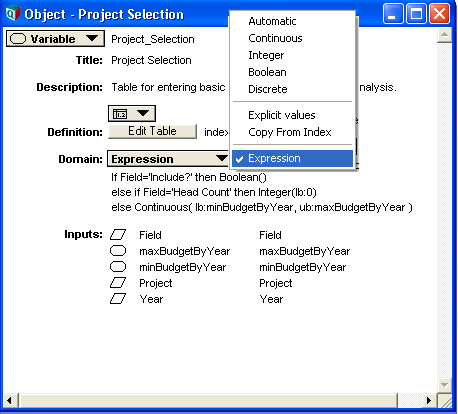# Domain Expressions

(Redirected from Boolean)

The domain of a variable specifies the space of possible values for each atomic cell of the result. Using the expression domain view, arbitrary Analytica expressions can be employed to compute this space:The computed domain may vary along one or more indexes and makes use of special domain specification functions (Continuous, Discrete, Integer, Boolean and Discrete). In the simplest case, a domain expression contains a single call to one of these functions, e.g.:

Continuous(lb: -1, ub: 1, nullOk: false)

More complex cases (such as in the above screenshot), arbitrary logic may be employed to compute the appropriate domain. The return value(s) for such an expression is typically a call to one of the 5 domain specification functions.

In addition to the 5 domain specification functions, a domain expression may return Null (for Automatic, meaning unspecified), or an unindexed list for a set of explicit values. In general, it is preferred to return explicit values using a call to Discrete, listing the possible values as parameters, e.g.:

Discrete('Democrat', 'Republican', 'Independent', 'Peace and Freedom', 'Green Party', 'Libertarian', 'Tea Party')

When the domain expression consists of a single identifier, this is taken to mean Copy from index, which copies the possible values from the index value of the indicted variable. This particular case is thus different from the behavior of the definition attribute that contains a single identifier, where the mid or prob value of the variable is returned. You can compute the domain within the definition of another variable, but to use it you would need to write the domain expression as: Mid(theComputedDomain), where theComputedDomain is the variable containing the computed domain expression in its definition.

The domain expression itself is always evaluated in mid mode.

Meta-inference algorithms that want to reason about the domain of variables may use the Domain Access Functions to obtain information about the space of possible values.

## Domain Specification Functions

### Boolean()

Boolean(nullOk)

Specifies that the only values allowed are 0, 1, and Null. If «nullOk» is specified as false, then the only allowed values are 0 and 1.

Boolean() is equivalent to Integer(0,1), so it is predominantly a convenience function.

Frontline solver engines, used by Analytica Optimizer, do distinguish between boolean and integer variables, so it is conceivable that the internal algorithms used to solve optimization problems may differ slightly when Integer(0,1) is used rather than Boolean().

### Continuous()

Continuous(lb, ub, zeroOk, nullOk)

Specifies a real-valued space of possible values. Optional lower and upper bounds («lb» and «ub») are used to identify a closed interval of the real-number line.

«zeroOk» is a boolean (true or false) indicating whether the value 0 is accepted. This is meaningful only when the value 0 is not in the [lb,ub] range. This is also known as a Semi-continuous variable.

Null values are considered acceptable by default unless the «nullOk» is specified as false.

Examples:

Continuous()
Continuous(lb: 0)
Continuous(0,1)
Continuous(lb: 1M, zeroOk: true)
Continuous(ub: 100, nullOk: false)

### Discrete()

Discrete(allowed..., type, nullOk)

Specifies that the space of possible values is discrete (i.e., not continuous). When the set of «allowed» values is unspecified, this is interpreted as meaning that the set of values is non-continuous, but the exact full set of possible values is unspecified (i.e., the Discrete option on the domain menu). When one or more «allowed» values is specified, this is interpreted as meaning that the listed values are the only values considered to be acceptable; however, Null is also considered to be acceptable unless «nullOk» is specified explicitly.

The «type» parameter, which must be used in named-parameter syntax, may be used to specify the acceptable data types for the discrete values. The parameter recognizes the following values: 'any', 'text', 'number', 'handle', and a subset of types can be specified by providing a list of these values to the parameter. The list-entry GUI will only operate when the type is limited to 'all', 'text' or 'number', but bounds checking and other facilities can make use of the other types.

Examples:

Discrete()
Discrete('item 1', 'item 2', 'item 3', 'item 4')
Discrete(type: 'number')
Discrete(type: ['number', 'text'])
Discrete(1, 2, 'n/a', nullOk: false)

### GroupedInteger()

GroupedInteger(groupName)

This domain type is intended specifically for a particular class of optimization problems. When a variable is of grouped-integer type, it means in any feasible solution, each scalar value belonging to the same «groupName» must have a value between 1 and N, where N is the total number of items in the same group, and all these scalar values must be different.

In a non-optimized case (such as just for bounds checking), the domain type is treated simply as equivalent to Integer(lb:1).

The «groupName» parameter can be omitted when an optimization problem contains only a single grouped-integer parameter. GroupedInteger() specifies the unnamed group, distinct from any groups that are named elsewhere.

Examples:

GroupedInteger()
GroupedInteger('Person')
GroupedInteger("C" & Column)

### Integer()

Integer(lb, ub, nullOk)

Specifies that the variable is integer-valued. A contiguous range of integers can be identifies by specifying the optional lower and upper bounds using the «lb» and «ub» parameters.

Null is also considered acceptable (i.e., won't trigger out-of-bounds warnings, etc.) unless «nullOk» is specified as false.

Examples:

Integer()
Integer(lb: 1)
Integer(lb: 0)
Integer(ub: -1)
Integer(lb: -180, ub: 180, nullOk: false)

## History

Introduced in Analytica 4.3.